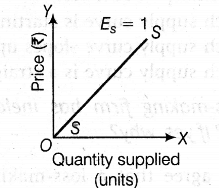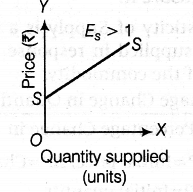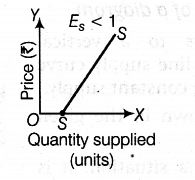# Explain the geometric method of measuring Price Elasticity of Supply

Explain the geometric method of measuring Price Elasticity of Supply (in case of straight line supply curve).

{{E}_{s}} = Intercept on the X- axis / Price at that Quantity.
(i) When a straight line positively sloped supply curve starts from the point of origin ‘O’, {{E}_{s}} = 1.
It is shown in the figure given below:(ii)When a straight line positively sloped supply curve starts from F-axis, {{E}_{s}},>1.It is shown in the figure given below:(in) When a straight line positively sloped supply curve starts from X-axis, {{E}_{s}},<1.
It is shown in the figure given below: